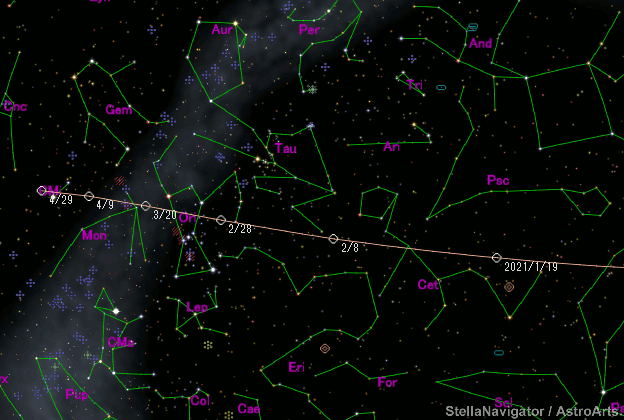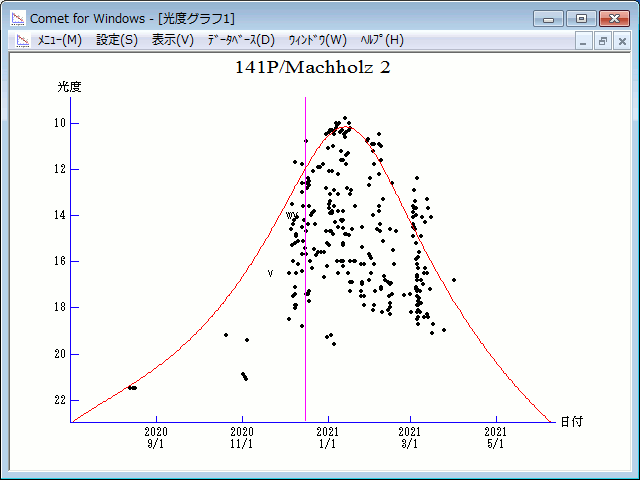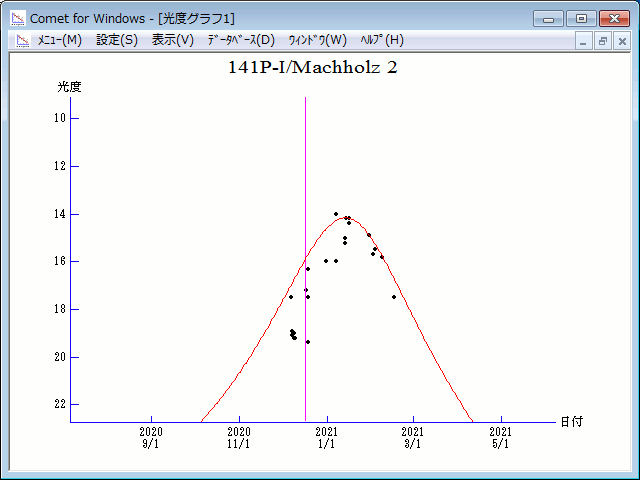# 141P/Machholz 2 (2020)###Pictures###Orbital Elements

```                       component A

Epoch 2022 Jan. 21.0 TT = JDT 2459600.5
T 2020 Dec. 16.31840 TT                                 Rudenko
q   0.8076519            (2000.0)            P               Q
n   0.18435827     Peri.  153.56479     +0.80363758     -0.55590222
a   3.0574613      Node   241.82747     +0.49438382     +0.82234835
e   0.7358423      Incl.   13.94634     +0.33128730     +0.12130920
P   5.35
From 1129 observations 2000 Jan. 3-2021 Mar. 24, mean residual 1".0.

outer component of 2020-2021 = component D in 1999 = component H in 2015

Epoch = 2020 Dec. 17.0 TT
T = 2020 Dec. 14.92239 TT        Peri. = 153.54047
e = 0.7355572                    Node  = 241.83987 2000.0
q = 0.8080285 AU                 Incl. =  13.94928
a =  3.0555893 AU   n = 0.18452771   P =   5.34 years

middle component = component I

Epoch = 2020 Dec. 17.0 TT
T = 2020 Dec. 15.84335 TT        Peri. = 153.53887
e = 0.7355697                    Node  = 241.84851 2000.0
q = 0.8079188 AU                 Incl. =  13.94450
a =  3.0553187 AU   n = 0.18455223   P =   5.34 years
```

###Finding Charts###Magnitudes Graph

```	Component A:
m1 = 13.5 + 5 log d + 22 log r(t - 25)

Component H:
m1 = 18.0 + 5 log d + 22 log r(t - 25)

Component I:
m1 = 17.5 + 5 log d + 22 log r(t - 25)
```##### The orbital elements of component A are published on M.P.E.C. 2022-K19. The orbital elements of component H and I are published on CBET 4924. The charts are made with StellaNavigator Ver.10 (AstroArts). The magnitudes graphs are made with Comet for Windows.